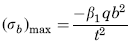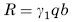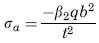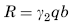Related Resources: calculators

### Load Uniformly Decreasing Flat Rectangular Plate Equation and Calculator

Flat Rectangular Plate, Two Edges Fixed, Two Edges Free Load Uniformly Decreasing from z = 0 to z = b Equation and Calculator.

Per. Roarks Formulas for Stress and Strain for flat plates with straight boundaries and constant thickness

Flat Rectangular plate, Two Edges Fixed, Two Edges Free Load Uniformly Decreasing from z = 0 to z = b.

 Rectangular plate; Two Edges Fixed Two Edges FreeLoad Uniformly Decreasing from z = 0 to z = bAt x = 0, z = 0
Stress and Reaction ForceReaction ForceStress and Reaction Force at x = 0, z = b if a = b, or Z = 0.6b if b/2 ≤ a < b. or z = 0.4b if a < b/2Reaction ForceWhere used:
E = Modulus of Elasticity (lbs/in2)
q = Total load or force to Plate (lbs/in2)
v = Poisson’s ratio (assumed to be 0.3)
t = plate thickness, (in)
a = plate length, (in)
b = plate width, (in)
σb = stress, (lbs/in2)
R = Reaction Force (lbs/in)
β1,2 = Constant From Table A
γ1,2 = Constant From Table A

Table A

 a/b 0.125 0.25 0.375 0.5 0.75 1 β1 0.043 0.133 0.212 0.328 0.537 0.695 β2 0.028 0.09 0.148 0.2 0.276 0.397 γ1 0.271 0.423 0.419 0.483 0.551 0.559 γ2 0.076 0.151 0.205 0.195 0.23 0.192

Reference:

Roarks Formulas for Stress and Strain, 7th Edition